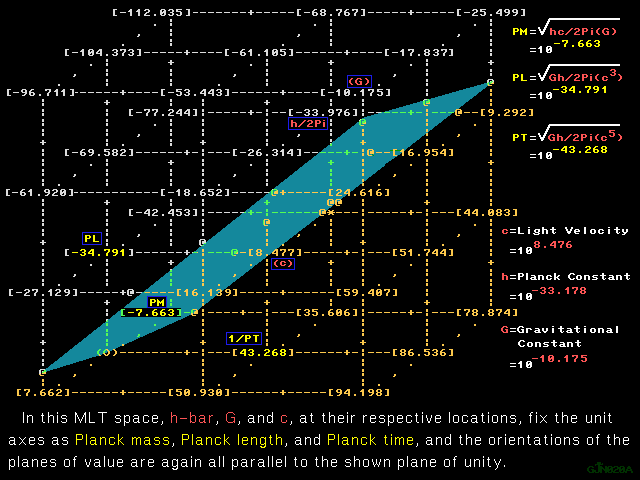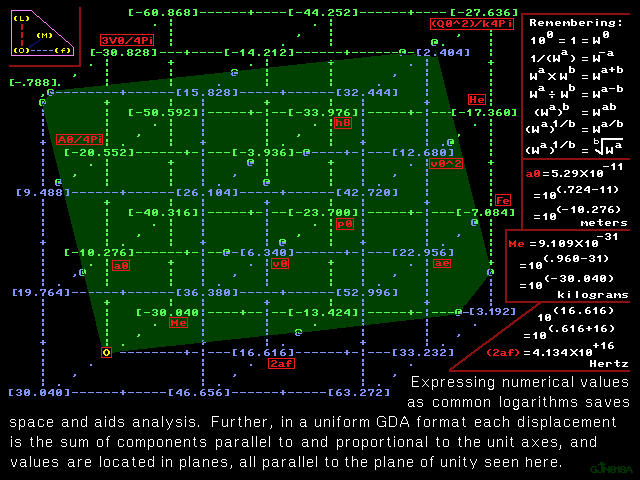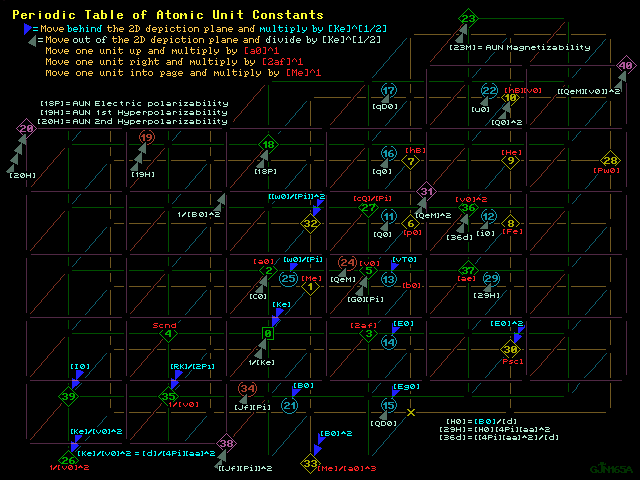```Color print      1280 X 960 GIF      Grayscale printColor print      1280 X 960 GIF      Grayscale print

Just as displacement on a one dimensional slide rule scale equates
to increasing or decreasing value of exponent and therefore equates
to multiplication or division, so is it true in these vector space
mappings that change of position corresponds to multiplication or
division by some multiple or sub-multiple of a unit or a quantity.

Remembering that [Ke] , the electrical interaction constant, is the
reciprocal of the atomic unit of permittivity: [Ke] = 1/([4Pi][k]),
each graphic is a 2D portrayal of an abstract 3D vector space, a 3D
map, but can include representation of a factor extending into the
4th dimension.  A wedge widening away from the viewer, extending
behind the 2D depiction plane and out of the 3D space equates above
so that two such wedges mean multiplication by [Meter/Farad]^1 while
each wedge widening toward the viewer, into the foreground, equates
division by [Meter/Farad]^1 is associated with two such wedges into
the foreground.

Wedges as displacement into the fourth dimension

In the portrayal below, mapping exact quantities called the atomic
units, a wedge widening away from the viewer and extending behind
the 2D depiction plane, out of the 3D space, is associated with a
multiplication by ([Ke]^[1/2]) = 1/{([4Pi][k])^[1/2]}, and every
two wedges widening toward the viewer into the foreground represent
multiplication by ([4Pi][k])^1 , the atomic unit of permittivity,
both numerically and in terms of units.

These conventions combine, conceptually above, and explicitly below,
with the mapping conventions confined entirely within the 3D space:

displace one unit distance to the right to multiply by [frequency]^1
and one unit distance to the left to divide by [frequency]^1 ,

displace one unit distance up to multiply by [length]^1
and one unit distance down to divide by [length]^1 ,

displace one unit distance "into the page" to multiply by [mass]^1
and one unit distance "out of the page" to divide by [mass]^1 ,
while still within the 3D space depicted in the 2D depiction plane,

mapping quantity calculus relationships among the atomic unit
fundamental constants, here is a periodic table of laws of physics.Color print      1280 X 960 GIF      B & W print

```